# 5. Filtering Data¶

Visualization can be characterized as a process of transforming raw data produced from experiments or simulations until it takes a form in which it can be interpreted and analysed. The visualization pipeline introduced in Section 1.2 formalizes this concept as a data flow paradigm where a pipeline is set up of sources, filters, and sinks (collectively called pipeline modules or algorithms). Data flows through this pipeline, being transformed at each node until it is in a form where it can be consumed by the sinks. In previous chapters, we saw how to ingest data into ParaView (Section 2) and how to display it in views (Section 4). If the data ingested into ParaView already has all the relevant attribute data, and it is in the form that can be directly represented in one the existing views, then that is all you would need. The true power of the visualization process, however, comes from leveraging the various visualization techniques such as slicing, contouring, clipping, etc., which are available as filters. In this chapter, we look at constructing pipelines to transform data using such filters.

## 5.1. Understanding filters¶

In ParaView, filters are pipeline modules or algorithms that have inputs and outputs. They take in data on their inputs and produce transformed data or results on their outputs. A filter can have multiple input and output ports. The number of input and output ports on a filter is fixed. Each input port accepts input data for a specific purpose or role within the filter. (E.g., the Resample With Dataset filter has two input ports. The one called Input is the input port through which the dataset providing the attributes to interpolate is ingested. The other, called Source , is the input port through which the dataset used as the mesh on which to re-sample is accepted.)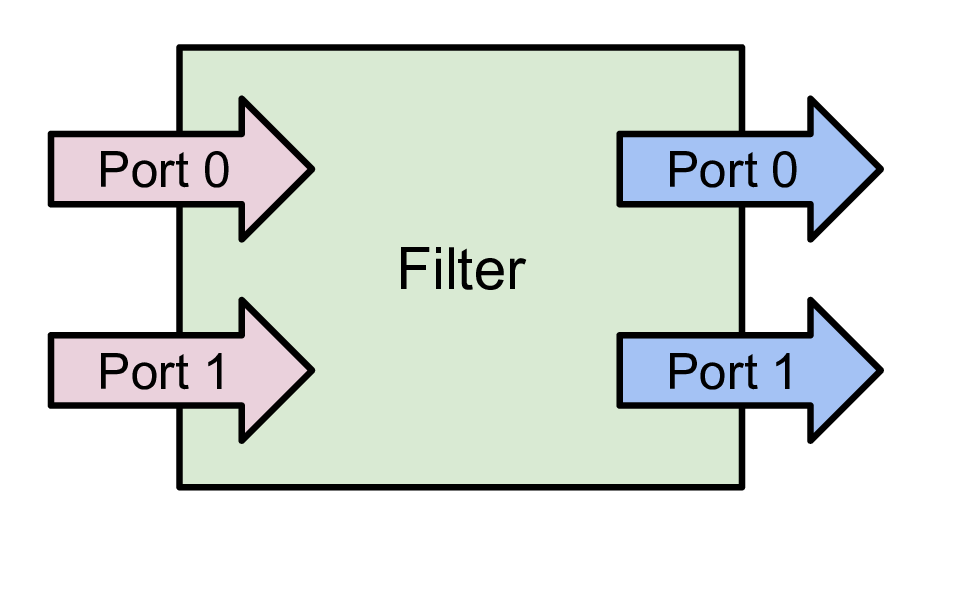Fig. 5.1 A filter is a pipeline module with inputs and outputs. Data enters a filter through the inputs. The filter transforms the data and produces the resulting data on its outputs. A filter can have one or more input and output ports. Each input port can optionally accept multiple input connections.

An input port itself can optionally accept multiple input connections, e.g., the Append Datasets filter, which appends multiple datasets to create a single dataset only has one input port (named Input ). However, that port can accept multiple connections for each of the datasets to be appended . Filters define whether a particular input port can accept one or many input connections.

Similar to readers, the properties on the filter allow you to control the filtering algorithm. The properties available depend on the filter itself.

## 5.2. Creating filters in paraview¶

All available filters in paraview are listed under the Filters menu. These are organized in various categories. To create a filter to transform the data produced by a source or a reader, you select the source in the Pipeline Browser to make it active, and then click on the corresponding menu item in the Filters menu. If a menu item is disabled, it implies that the active source does not produce data that can be transformed by this filter.

Did you know?

If a menu item in the Filters menu is disabled, it implies that the active source(s) is not producing data of the expected type or the characteristics needed by the filter. On Windows and Linux machines, if you hover over the disabled menu item, the status bar will show the reason why the filter is not available.

### 5.2.1. Multiple input connections¶

When you create a filter, the active source is connected to the first input port of the filter. Filters like Append Datasets can take multiple input connections on that input port. In such a case, to pass multiple pipeline modules as connections on a single input port of a filter, select all the relevant pipeline modules in the Pipeline Browser . You can select multiple items by using the CTRL (or ) and key modifiers. When multiple pipeline modules are selected, only the filters that accept multiple connections on their input ports will be enabled in the Filters menu.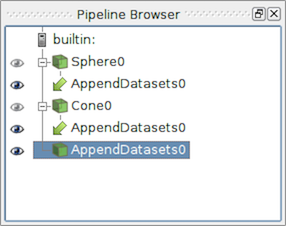Fig. 5.2 The Pipeline Browser showing a pipeline with multiple input connections. The Append Datasets filter has two input connections on its only input port, Sphere0 and Cone0 .

### 5.2.2. Multiple input ports¶

Most filters have just one input port. Hence, as soon as you click on the filter name in the Filters menu, it will create a new filter instance and that will show up in the Pipeline Browser . Certain filters, such as Resample With Dataset , have multiple inputs that must be set up before the filter can be created. In such a case, when you click on the filter name, the Change Input Dialog will pop up, as seen in Fig. 5.3. This dialog allows you to select the pipeline modules to be connected to each of the input ports. The active source(s) is connected by default to the first input port. You are free to change those as well.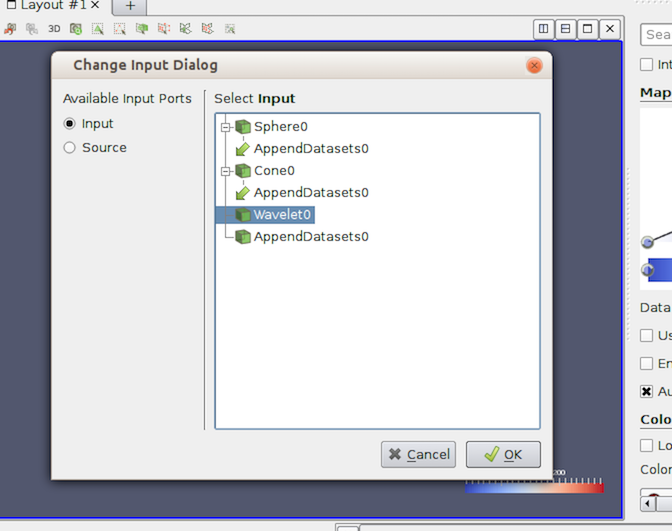Fig. 5.3 The Change Input Dialog is shown to allow you to pick inputs for each of the input ports for a filter with multiple input ports. To use this dialog, first select the Input Port you want to edit on the left side, and select the pipeline module(s) that are to be connected to this input port. Repeat the step for the other input port(s). If an input port can accept multiple input connections, you can select multiple modules, just like in the Pipeline Browser .

### 5.2.3. Changing input connections¶

paraview allows you to change the inputs to a filter after the filter has been created. To change inputs to a filter, right-click on the filter in the Pipeline Browser to get the context menu, and then select Change Input... . This will pop up the same Change Input Dialog as when creating a filter with multiple input ports. You can use this dialog to set new inputs for this filter.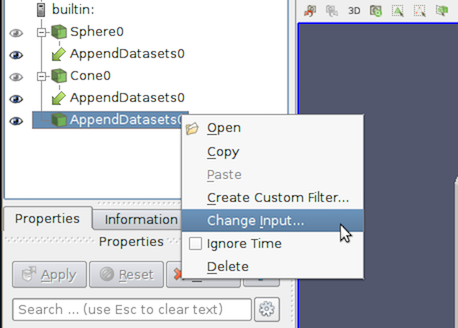Fig. 5.4 The context menu in the Pipeline Browser showing the option to change inputs for a filter.

Did you know?

While the Filters menu is a handy way to create new filters, with the long list of filters available in ParaView, manually finding a particular filter in this menu can be very challenging. To make it easier, ParaView incorporates a quick launch mechanism. When you want to create a new filter (or a source), simply type CTRL + Space or Alt + Space. This will pop up the quick-launch dialog. Now, start typing the name of the filter you want. As you type, the dialog will update to show the filters and sources that match the typed text. You can use the arrow keys to navigate and use the Enter key to create the selected filter (or source). Note that filters may be disabled, as was the case in the Filters menu but by default the selected item will be the first enabled filter. You can use Esc to clear the text you have typed so far. Hit the Esc a second time, and the dialog will close without creating any new filter. You can also display the quick-launch dialog using CTRL + + Space, Alt + + Space, or + + Space. In this case, the selected filter will be automatically applied once created i.e. equivalent to creating the filter and then clicking the Apply button.

## 5.3. Creating filters in pvpython¶

To create a filter in pvpython, you simply create the object by using its name as a constructor function.

>>> from paraview.simple import *
...
>>> filter = Shrink()


Similar to paraview, the filter will use the active source(s) as the input. Additionally, you can explicitly specify the input in the function arguments.

>>> reader = OpenDataFile(...)
...


### 5.3.1. Multiple input connections¶

To setup multiple input connections, you can specify the connections as follows:

>>> sphere = Sphere()
>>> cone = Cone()

# Simply pass the sources as a list to the constructor function.
>>> appendDatasets = AppendDatasets(Input=[sphere, cone])
>>> print(appendDatasets.Input)
[<paraview.servermanager.Sphere object at 0x6d75f90>, <paraview.servermanager.Cone object at 0x6d75c50>]


### 5.3.2. Multiple input ports¶

Setting up connections to multiple input ports is similar to the multiple input connections, except that you need to ensure that you name the input ports properly.

>>> sphere = Sphere()
>>> wavelet = Wavelet()

>>> resampleWithDataSet = ResampleWithDataset(Input=sphere, Source=wavelet)


### 5.3.3. Changing input connections¶

Changing inputs in Python is as simple as setting any other property on the filter.

# For filter with single input connection
>>> shrink.Input = cone

# for filters with multiple input connects

# to add a new input.
>>> appendDatasets.Input.append(sphere)

# to change multiple ports
>>> resampleWithDataSet.Input = wavelet2
>>> resampleWithDataSet.Source = cone


## 5.4. Changing filter properties in paraview¶

Filters provide properties that you can change to control the processing algorithm employed by the filter. Changing and viewing properties on filters is the same as with any other pipeline module, including readers and sources. You can view and change these properties, when available, using the Properties panel. Section 1 covers how to effectively use the Properties panel. Since this panel only shows the properties present on the active source , you must ensure that the filter you are interested in is active. To make the filter active, use the Pipeline Browser to click on the filter and select it.

## 5.5. Changing filter properties in pvpython¶

With pvpython, the available properties are accessible as properties on the filter object, and you can get or set their values by name (similar to changing the input connections (Section 5.3.3)).

# You can save the object reference when it's created.
>>> shrink = Shrink()

>>> Shrink() # <-- this will make the Shrink the active source.
>>> shrink = GetActiveSource()

# To figure out available properties, you can always use help.
>>> help(shrink)
Help on Shrink in module paraview.servermanager object:

class Shrink(SourceProxy)
|  The Shrink filter
|  causes the individual cells of a dataset to break apart
|  from each other by moving each cell\'s points toward the
|  centroid of the cell. (The centroid of a cell is the
|  average position of its points.) This filter operates on
|  any type of dataset and produces unstructured grid
|  output.
|  ----------------------------------------------------------------------
|  Data descriptors defined here:
|
|  Input
|      This property specifies the input to the Shrink
|      filter.
|
|  ShrinkFactor
|      The value of this property determines how far the points
|      will move. A value of 0 positions the points at the centroid of the
|      cell; a value of 1 leaves them at their original
|      positions.
....

# To get the current value of a property:
>>> sf = shrink.ShrinkFactor
>>> print sf
0.5

# To set the value
>>> shrink.ShrinkFactor = 0.75


In the rest of this chapter, we will discuss some of the commonly used filters in detail. They are grouped under categories based on the type of operation that they perform.

## 5.6. Filters for sub-setting data¶

These filters are used for extracting subsets from an input dataset. How this subset is defined and how it is extracted depends on the type of the filter.

### 5.6.1. Clip¶

Clip is used to clip any dataset using either an implicit function (such as a plane, sphere, or a box) or using values of a scalar data array in the input dataset. A scalar array is a point or cell attribute array with a single component. Clipping involves iterating over all cells in the input dataset and then removing those cells that are considered outside of the space defined by the implicit function or that have an attribute values less than the selected value. For cells that straddle the clipping surface, these are clipped to pass through the part of the cell that is truly inside the specified implicit function (or greater than the scalar value).

This filter converts any dataset into an unstructured grid (Section 3.1.7) or a multi-block of unstructured grids (Section 3.1.10) in the case of composite datasets.

#### 5.6.1.1. Clip in paraview¶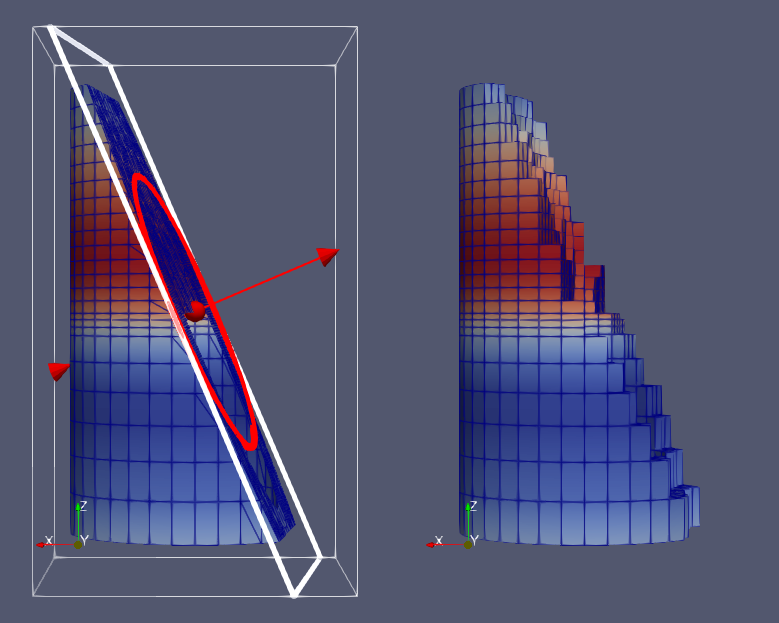Fig. 5.5 Comparison between results produced by the Clip filter with Crinkle Clip unchecked (left) and checked (right) when clipping with an implicit plane. The image on the left also shows the 3D widget used to interactivly place the implicit plane for the clipping operation.

To create the Clip filter, you can use the Filters > Common or the Filters > Alphabetical menu. This filter is also accessible from the Common filters toolbar. You can click thebutton to create this filter.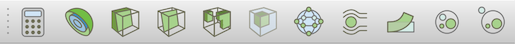Fig. 5.6 The Common filters toolbar in paraview for quick access to the commonly used filters.

On the Properties panel, you will see the available properties for this filter. One of the first things that you should select is the Clip Type . Clip Type is used to specify the type of implicit function to use for the clipping operations. The available options include Plane , Box , Sphere , and Scalar . Selecting any one of these options will update the panel to show properties that are used to define the implicit function, e.g., the Origin and the Normal for the Plane or the Center and the Radius for the Sphere . If you select Scalar , the panel will let you pick the data array and the value with which to clip. Remember, cells with the data value greater than or equal to the selected value are considered in and are passed through the filter.

Did you know?

When clipping with implicit functions, ParaView renders widgets in the active view that you can use to interactively control the implicit function, called 3D widgets . As you interact with the 3D widget, the panel will update to reflect the current values. The 3D widget is considered as an aid and not as a part of the actual visualization scene. Thus, if you change the active source and the Properties panel navigates away from this filter, the 3D widget will automatically be hidden.

The Inside Out option can be used to invert the behavior of this filter. Basically, it flips the notion of what is considered inside and outside of the given clipping space.

Check Crinkle Clip if you don’t want this filter to truly clip cells on the boundary, but want to preserve the input cell structure and to pass the entire cell on through the boundary (Fig. 5.5). This option is not available when clipping by Scalar .

#### 5.6.1.2. Clip in pvpython¶

This following script demonstrates various aspects of using the Clip filter in pvpython.

# Create the Clip filter.
>>> clip = Clip(Input=...)

# Specify a 'ClipType' to use.
>>> clip.ClipType = 'Plane'

# You can also use the SetProperties API instead.
>>> SetProperties(clip, ClipType='Plane')

>>> print(clip.GetProperty('ClipType').GetAvailable())
['Plane', 'Box', 'Sphere', 'Scalar']

# To set the plane origin and normal
>>> clip.ClipType.Origin = [0, 0, 0]
>>> clip.ClipType.Normal = [1, 0, 0]

# If you want to change to Sphere and set center and
# radius, you can do the following.
>>> clip.ClipType = 'Sphere'
>>> clip.ClipType.Center = [0, 0, 0]

# Using SetProperties API, the same looks like
>>> SetProperties(clip, ClipType='Sphere')
>>> SetProperties(clip.ClipType, Center=[0, 0, 0],

# To set Crinkle clipping.
>>> clip.Crinkleclip = 1

# For clipping with scalar, you pick the scalar array
# and then the value as follows:
>>> clip.ClipType = 'Scalar'
>>> clip.Scalars = ('POINTS', 'Temp')
>>> clip.Value = 100

# As always, to get the list of available properties on
# the clip filter, use help()
>>> help(clip)
Help on Clip in module paraview.servermanager object:

class Clip(SourceProxy)
|  The Clip filter
|  cuts away a portion of the input dataset using an
|  implicit plane. This filter operates on all types of data
|  sets, and it returns unstructured grid data on
|  output.
|
|  ----------------------------------------------------------------------
|  Data descriptors defined here:
|
|  ClipType
|      This property specifies the parameters of the clip
|      function (an implicit plane) used to clip the dataset.
|
|  Crinkleclip
|      This parameter controls whether to extract entire cells
|      in the given region or clip those cells so all of the output one stay
|      only inside that region.
|
|  Input
|      This property specifies the dataset on which the Clip
|      filter will operate.
|
|  InsideOut
|      If this property is set to 0, the clip filter will
|      return that portion of the dataset that lies within the clip function.
|      If set to 1, the portions of the dataset that lie outside the clip
|      function will be returned instead.
...

# To get help on a specific implicit function type, make it the active
# ClipType and then use help()
>>> clip.ClipType = 'Plane'
>>> help(clip.ClipType)
Help on Plane in module paraview.servermanager object:

class Plane(Proxy)
...


Common Errors

It is very easy to forget that clipping a structured dataset such as image data can dramatically increase the memory requirements, since this filter will convert the structured dataset into an unstructured grid due to the nature of the clipping operation itself. For structured dataset, think about using Slice or Extract Subset filters instead, whenever appropriate. Those are not entirely identical operations, but they are often sufficient.

### 5.6.2. Slice¶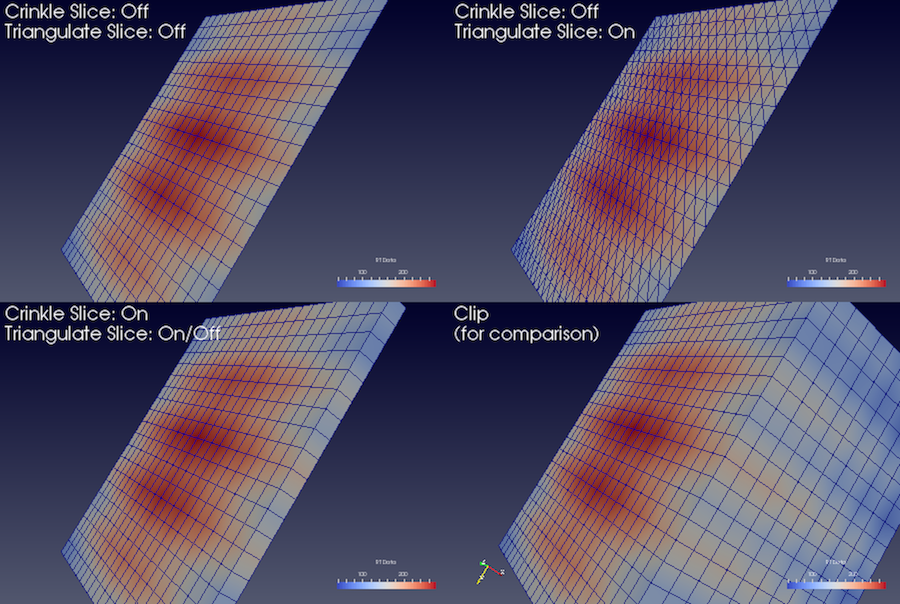Fig. 5.7 Comparison between results produced by the Slice filter when slicing image data with an implicit plane with different options. The lower-left image shows the output produced by the Clip filter when clipping with the same implicit function, for contrast.

The Slice filter slices through the input dataset with an implicit function such as a plane, a sphere, or a box. Since this filter returns data elements along the implicit function boundary, this is a dimensionality reducing filter (except when crinkle slicing is enabled), i.e., if the input dataset has 3D elements like tetrahedrons or hexahedrons, the output will have 2D elements, line triangles, and quads, if any. While slicing through a dataset with 2D elements, the result will be lines.

The properties available on this filter, as well as the way of setting this filter up, is very similar to the Clip filter with a few notable differences. What remains similar is the set up of the implicit function – you have similar choices: Plane , Box , Sphere , and Cylinder , as well as the option to toggle Crinkle slice (i.e., to avoid cutting through cells, pass complete cells from the input dataset that intersects the implicit function).

What is different includes the lack of slicing by Scalar (for that, you can use the Contour filter) and a new option, Triangulate the slice . Fig. 5.7 shows the difference in the generated meshes when various slice properties are changed.

The Slice filter is more versatile than the Slice representation. First, the Slice representation is available for image datasets only, whereas the Slice filter can be used on any type of 3D dataset. Second, the representation extracts a subset of the image consisting of a 2D slice oriented in the XY, YZ, or XZ planes at the image voxel locations while the plane used by the filter can be placed arbitrarily. Third, since the Slice representation always shows a flat object and lighting may interfere with interpretation of data values on the slice, lighting is not applied to the Slice representation. Lighting is applied, however, to results from the Slice filter. Lastly, the Slice representation may be faster than the filter to update and scrub through different slices because it does not need to compute the intersection of a plane with cells in the dataset.

In paraview, this filter can be created using thebutton on the Common filters toolbar, besides the Filters menu.

### 5.6.3. Extract Subset¶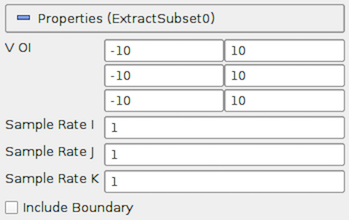Fig. 5.8 The Properties panel for the Extract Subset filter showing all available properties (including the advanced properties).

For structured datasets such as image datasets (Section 3.1.3), rectilinear grids (Section 3.1.4), and curvilinear grids (Section 3.1.5), Extract Subset filter can be used to extract a region of interest or a subgrid. The region to extract is specified using structured coordinates, i.e., the $$i$$, $$j$$, $$k$$ values. Whenever possible, this filter should be preferred over Clip or Slice for structured datasets, since it preserves the input data type. Besides extracting a subset, this filter can also be used to resample the dataset to a coarser resolution by specifying the sample rate along each of the structured dimensions.

#### 5.6.3.1. Extract Subset in paraview¶

This is one of the filters available on the Common filters toolbarTo specify the region of interest, use the VOI property. The values are specified as min and max values for each of the structured dimensions ($$i$$, $$j$$, $$k$$,) in each row. Sample Rate I , Sample Rate J , and Sample Rate K specify the sub-sampling rate. Set it to a value greater than one to sub-sample. Include Boundary is used to determine if the boundary slab should be included in the extracted result, if the sub-sampling rate along that dimension is greater than 1, and the boundary slab would otherwise have been skipped.

### 5.6.4. Threshold¶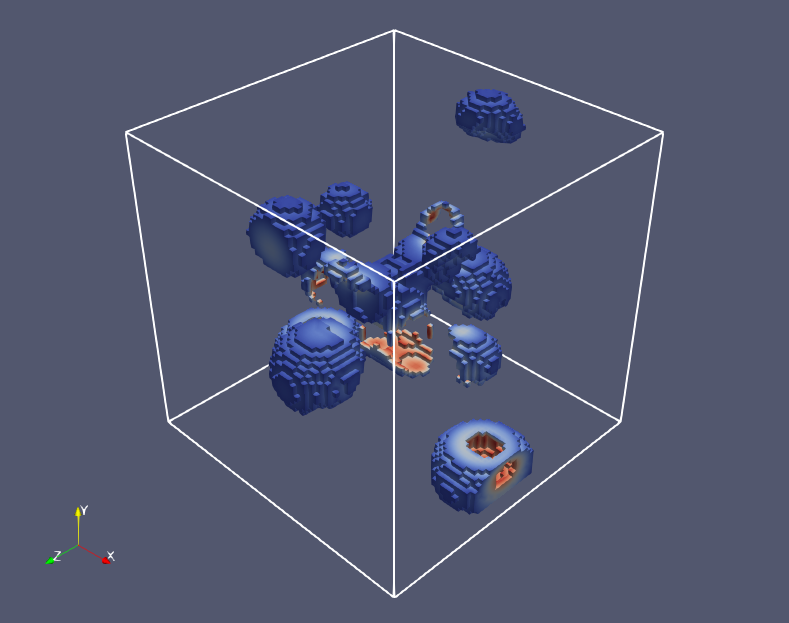Fig. 5.9 Results from using the Threshold filter on the iron_protein.vtk dataset from ParaView data.

The Threshold filter extracts the portions of the input dataset whose scalars lie within the specified range. This filter operates on either point-centered or cell-centered data. This filter operates on any type of dataset and produces an unstructured grid output. To use this filter, you select the Scalars with which to threshold, and then specify the data range that selects which cells are included in the output. When thresholding with cell data, all cells that have scalars within the specified range will be passed through the filter. When thresholding with point data, cells with all points with scalar values within the range are passed through if All Scalars is checked; otherwise, cells with any point that passes the thresholding criteria are passed through.

#### 5.6.4.1. Threshold in paraview¶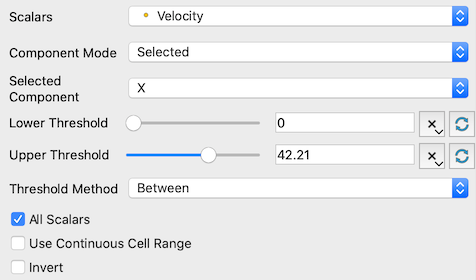Fig. 5.10 The Properties panel for the Threshold filter.

This filter is represented ason the Common filters toolbar. After selecting the Scalars with which to threshold from the combo-box, you select the Minimum and Maximum values to specify the range. If the range shown by the sliders is not sufficient, you can manually type the values in the input boxes. The values are deliberately not clamped to the current data range. The Scalars combo-box uses icons to help you differentiate between point data arraysand cell data arrays#### 5.6.4.2. Threshold in pvpython¶

# Create the filter. If Input is not specified, the active source will be
# used as the input.
>>> threshold = Threshold(Input=...)

# Here's how to select a scalar array.
>>> threshold.Scalars = ("POINTS", "scalars")

# The value is a tuple with first value as the association:
# either "POINTS" or "CELLS" and the second value is the name of the selected
array.

>>> print(threshold.Scalars)
['POINTS', 'scalars']

>>>> print(threshold.Scalars.GetArrayName())
'scalars'

>>> print(threshold.Scalars.GetAssociation())
'POINTS'

# The threshold range is specified as:
>>> threshold.ThresholdRange = [63.75, 252.45]


To determine the types of arrays available in the input dataset, and their ranges, refer to the discussion on data information in Section 3.3.

### 5.6.5. Iso Volume¶

The Iso Volume filter is similar to Threshold in that you use this to create an output dataset from an input where the cells that satisfy the specified range are scalar values. In fact, the filter is identical to Threshold when the cell data scalars are selected. For point data scalars, however, this filter acts similar to the Clip filters when clipping with scalars, in that cells are clipped along the iso-surface formed by the scalar range.

### 5.6.6. Extract Selection¶

Extract Selection is a general-purpose filter to extract selected elements from a dataset. There are several ways of making selections in ParaView. Once you have made the selection, this filter allows you to extract the selected elements as a new dataset for further processing. We will cover this filter in more detail when looking at selections in ParaView in Section 6.6.

## 5.7. Filters for geometric manipulation¶

These filters are used to transform the geometry of the dataset without affecting its topology or its connectivity.

### 5.7.1. Transform¶

The Transform can be used to arbitrarily translate, rotate, and scale a dataset. The transformation is applied by scaling the dataset, rotating it, and then translating it based on the values specified.

As this is a geometric manipulation filter, this filter does not affect connectivity in the input dataset. While it tries to preserve the input dataset type, whenever possible, there are cases when the transformed dataset can no longer be represented in the same data type as the input. For example, with image data (Section 3.1.3) and rectilinear grids (Section 3.1.4) that are transformed by rotation, the output dataset can be non-axis aligned and, hence, cannot be represented as either data types. In such cases, the dataset is converted to a structured, or curvilinear, grid (Section 3.1.5). Since curvilinear grids are not as compact as the other two, the need to store the results in a more general data type implies a considerable increase in the memory footprint.

### 5.7.2. Transform in paraview¶

You can create a new Transform from the Filters > Alphabetical menu. Once created, you can set the transform as the translation, rotation, and scale to use utilizing the Properties panel. Similar to Clip , this filter also supports using a 3D widget to interactively set the transformation.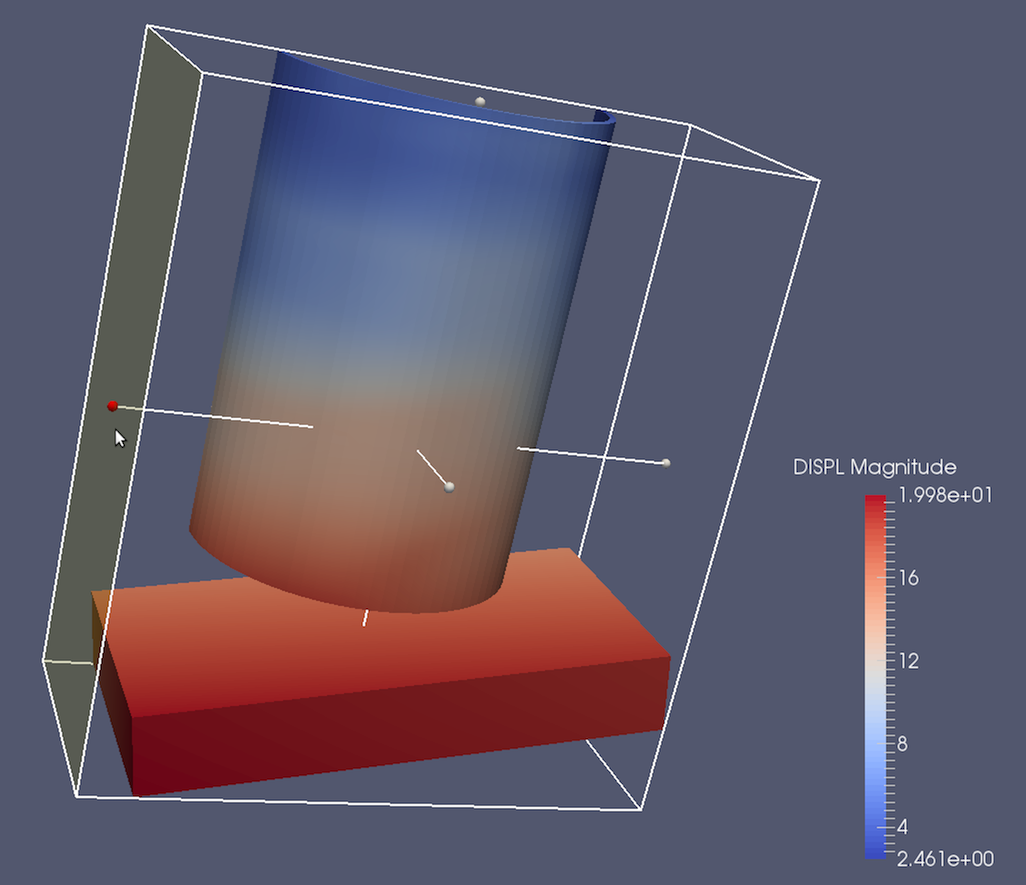Fig. 5.11 The Transform filter showing the 3D widget that can be used to interactively set the transform.

### 5.7.3. Transform in pvpython¶

# To create the filter(if Input is not specified, the active source will be
# used as the input).
>>> transform = Transform(Input=...)

# Set the transformation properties.
>>> transform.Translate.Scale = [1, 2, 1]
>>> transform.Transform.Translate = [100, 0, 0]
>>> transform.Transform.Rotate = [0, 0, 0]


### 5.7.4. Reflect¶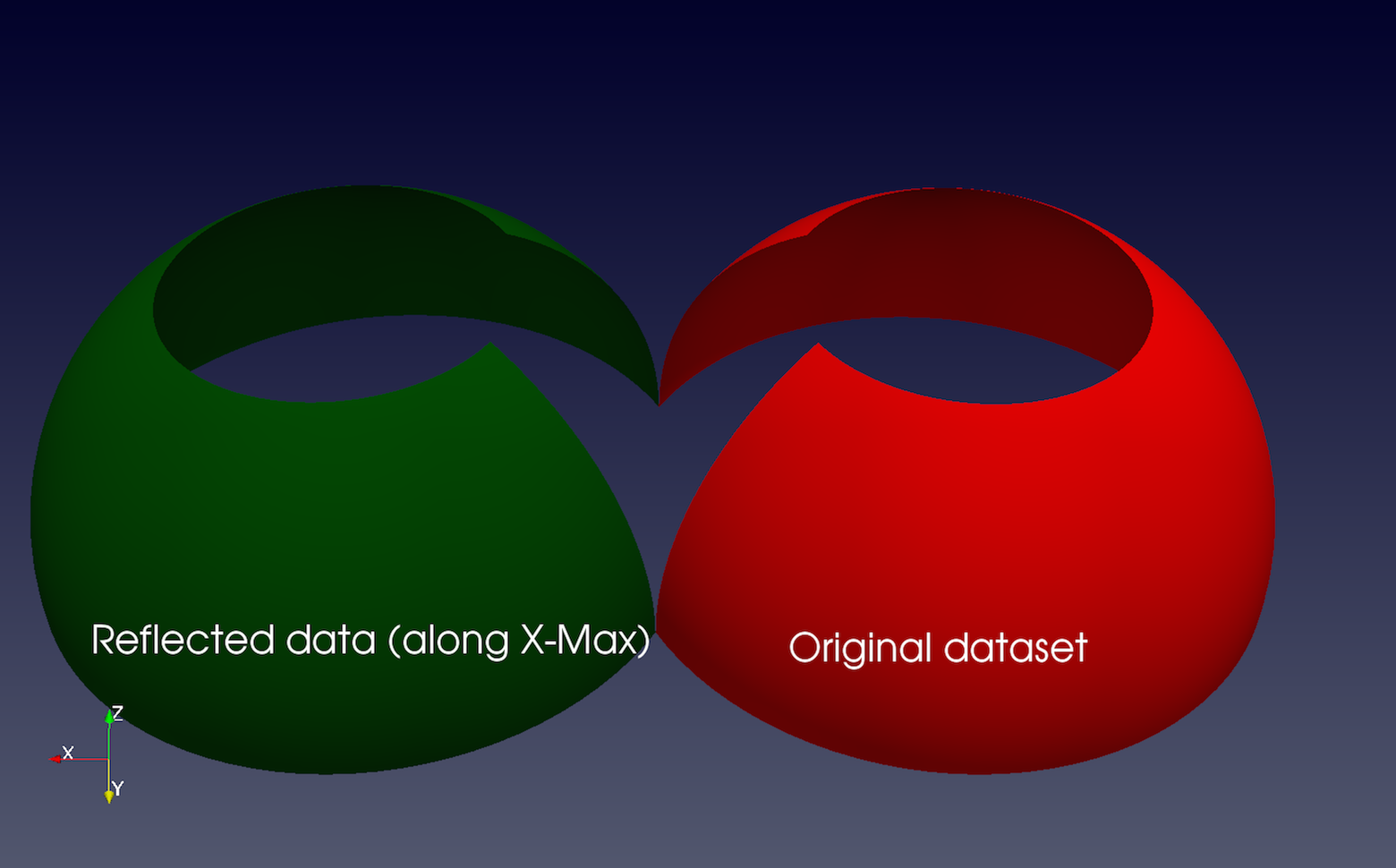Fig. 5.12 The Reflect filter can be used to reflect a dataset along a specific axis plane.

Reflect can be used to reflect any dataset across an axis plane. You can pick the axis plane to be one of the planes formed by the bounding box of the dataset. For that, set Plane as X Min , X Max , Y Min , Y Max , Z Min , or Z Max . To reflect across an arbitrary axis plane, select X , Y , or Z for the Plane property, and then set the Center to the plane offset from the origin.

This filter reflects the input dataset and produces an unstructured grid (Section 3.1.7). Thus, the same caveats for Clip and Threshold filter apply here when dealing with structured datasets.

### 5.7.5. Warp By Vector¶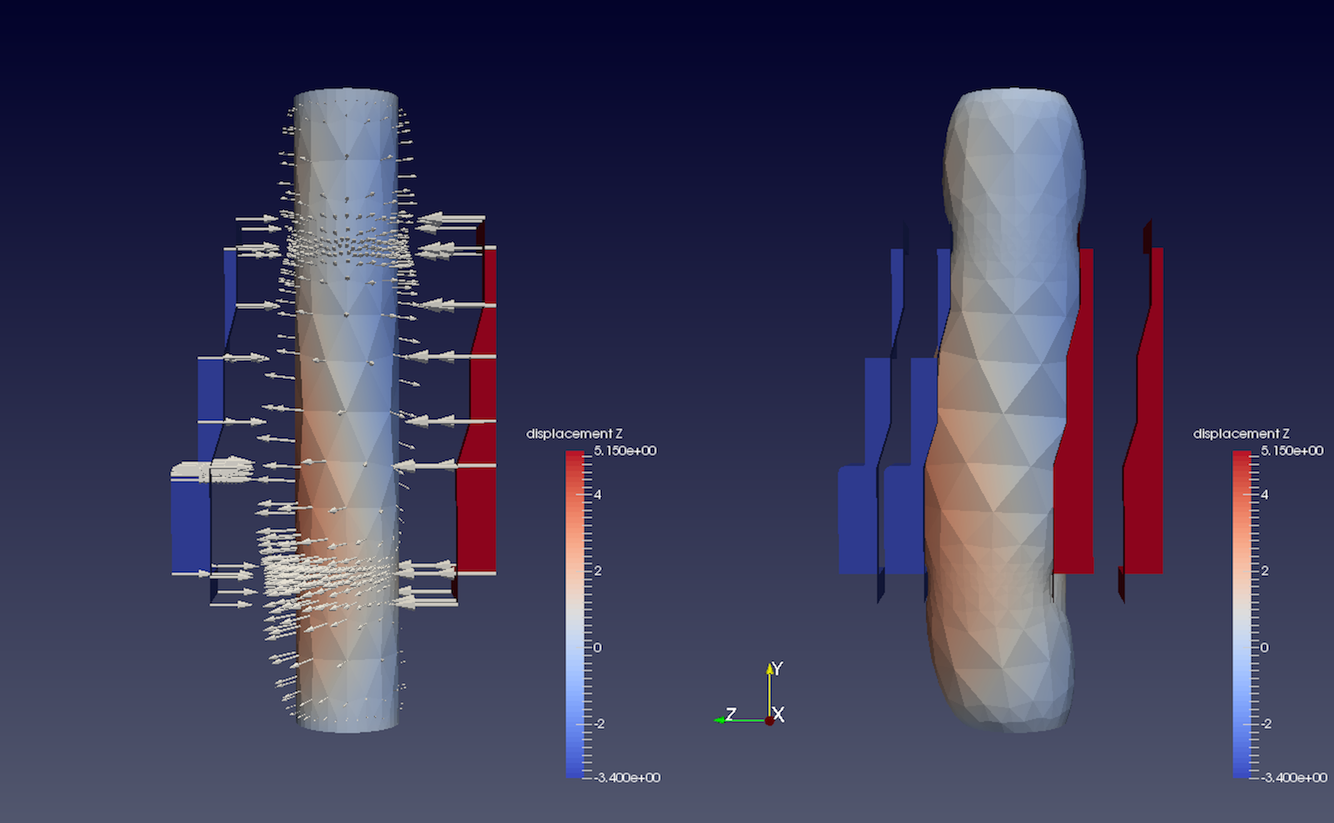Fig. 5.13 The Warp By Vector filter can be used to displace points in original data shown on the left, using the displacement vectors (indicated by arrow glyphs Section 5.8.1) to produce the result shown on the right.

Warp By Vector can be used to displace point coordinates in an input mesh using vectors in the dataset itself. You select the vectors to use utilizing the Vectors property on the Properties panel. Scale Factor can be used to scale the displacement applied.

### 5.7.6. Warp By Scalar¶

Warp By Scalar is similar to Warp By Vector in the sense that it warps the input mesh. However, it does so using a scalar array in the input dataset. The direction of displacement can either be explicitly specified using the Normal property, or you can check Use Normal to use normals at the point locations.

## 5.8. Filters for sampling¶

These filters compute new datasets that represent some essential features from the datasets that they take as input.

### 5.8.1. Glyph¶

Glyph is used to place markers or glyphs at point locations in the input dataset. The glyphs can be oriented or scaled based on vector and scalar attributes on those points.

To create this filter in paraview, you can use the Filters menu, as well as thebutton on the Common filters toolbar. You first select the type of glyph using one of the options in Glyph Type . The choices include Arrow , Sphere , Cylinder , etc. Next, you select the point arrays to use as the Orientation Array (selecting No orientation array will result in the glyphs not being oriented). Similarly, you select a point array to serve as the glyph Scale Array (no scaling is performed if No scale array is chosen).

If the Scale Array is set to a vector array, the Vector Scale Mode property is available to select which properties of the vector should be used to transform each glyph. If Scale by Magnitude is chosen, then the glyph at a point will be scaled by the magnitude of the vector at that point. If Scale by Components is chosen, glyphs will be scaled separately in each dimension by the vector component in that dimension.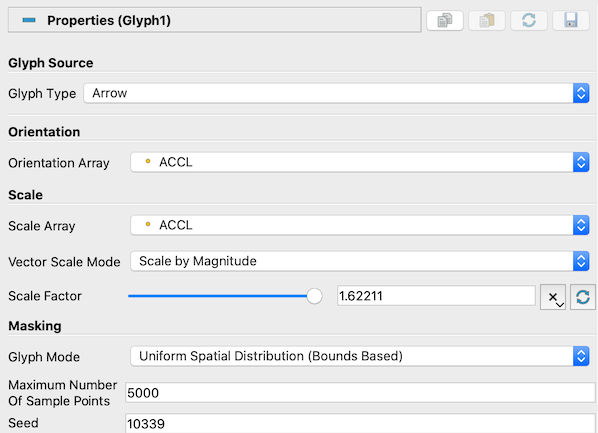Fig. 5.14 The Properties panel for the Glyph filter.

The Scale Factor is used to apply a constant scaling to all the glyphs, independent of the Scale Array and Vector Scale Mode properties. Choosing a good scale factor depends on several things including the bounds on the input dataset, the Scale Array and Vector Scale Mode selected, and the range for the array selected as the Scale Array . You can use thebutton next to the Scale Factor widget to have paraview pick a usually reasonable scale factor value based on the current dataset and scaling properties.

The Masking properties control which points from the input dataset get glyphed. The Glyph Mode controls how points are selected to be glyphs (Fig. 5.15). The available options are as follows:

• All Points : This selects all points in the input dataset for glyphing. Use this mode with caution and only when the input dataset has relatively few points. Since all points in the input dataset are glyphed, this can not only cause visual clutter, but also clog up memory and take a long to time to generate and render the glyphs.

• Every Nth Points : This elects every $$n^{th}$$ point in the input dataset for glyphing, where $$n$$ can be specified using Stride . Setting Stride to 1 will have the same effect as All Points .

• Uniform Spatial Distribution : This selects a random set of points. The algorithm works by first computing up to Maximum Number of Sample Points in the space defined by the bounding box of the input dataset. Then, points in the input dataset that are close to the point in this set of sample points are glyphed. The Seed is used to seed the random number generator used to generate the sample points. This ensures that the random sample points are reproducible and consistent.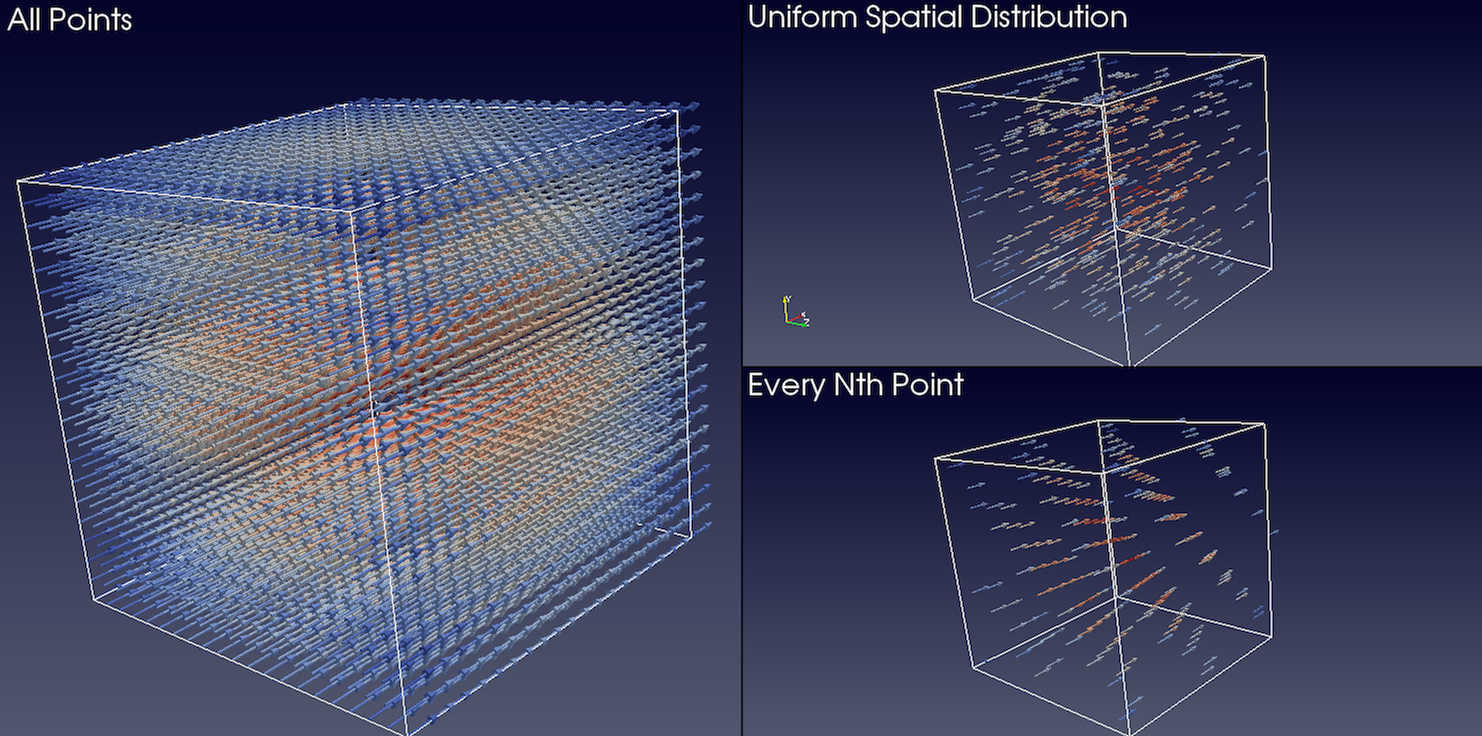Fig. 5.15 Comparison between various Glyph Mode s when applied to the same dataset generated by the Wavelet source.

Did you know?

The Glyph representation can be used for many of the same visualizations where a Glyph filter might be used. It may offer faster rendering and consume less memory than the Glyph filter with similar capabilities. In circumstances where generating a 3D geometry is required, e.g., when exporting glyph geometry to a file, the Glyph filter is required.

### 5.8.2. Glyph With Custom Source¶

Glyph With Custom Source is the same as Glyph , except that instead of a limited set of Glyph Type , you can select any data source producing a polygonal dataset (Section 3.1.8) available in the Pipeline Browser . To use this filter, select the data source you wish to glyph in the Pipeline Browser and attach this filter to it. You will be presented a dialog where you can set the Input (which defaults to the source you selected) and the Glyph Source .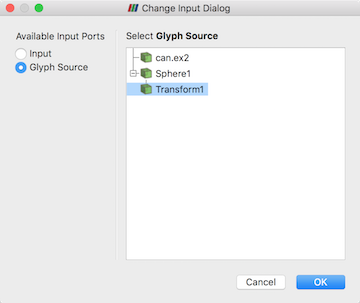Fig. 5.16 Setting the Input and Glyph Source in the Glyph With Custom Source filter.

### 5.8.3. Stream Tracer¶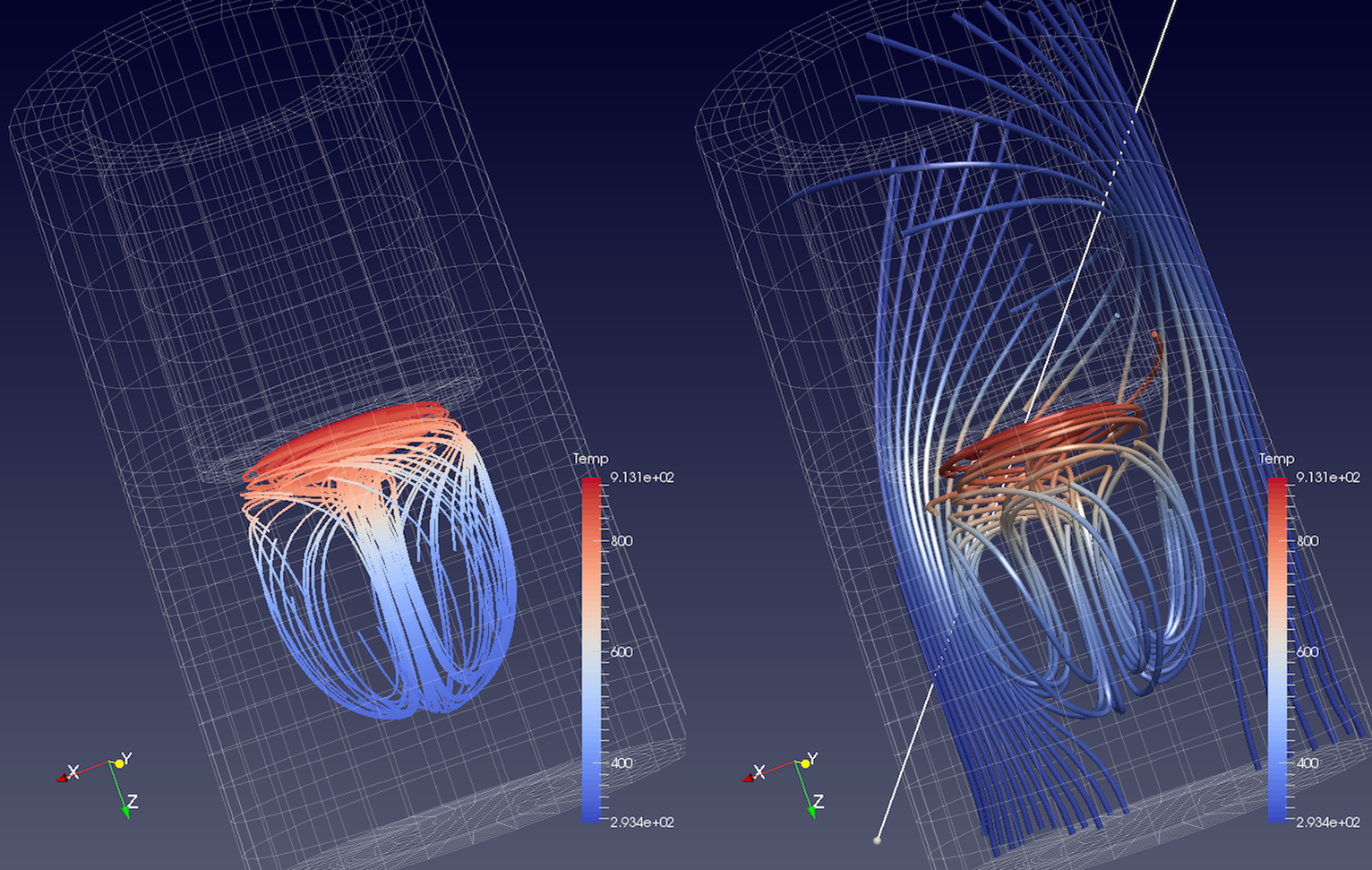Fig. 5.17 Streamlines generated from the disk_out_ref.ex2 dataset using the Point Source (left) and the High Resolution Line Source (right). On the left, we also added the Tube filter to the output of the Stream Tracer filter to generate 3D tubes rather than 1D polygonal lines, which can be hard to visualize due to lack of shading.

The Stream Tracer filter is used to generate streamlines for vector fields. In visualization, streamlines refer to curves that are instanteneously tangential to the the vector field in the dataset. They provide an indication of the direction in which the particles in the dataset would travel at that instant in time. The algorithm works by taking a set of points, known as seed points, in the dataset and then integrating the streamlines starting at these seed points.

In paraview, you can create this filter using the Filters menu, as well as thebutton on the Common filters toolbar. To use this filter, you first select the attribute array to use as the Vectors for generating the streamline. Integration Parameters let you fine tune the streamline integration by specifying the direction to integrate, Integration Direction , as well as the type of integration algorithm to use, Integrator Type . Advanced integration parameters are available in the advanced view of the Properties panel that let you further tune the integration, including specifying the step size and others. You use the Maximum Streamline Length to limit the maximum length for the streamline – the longer the length, the longer the generated streamlines.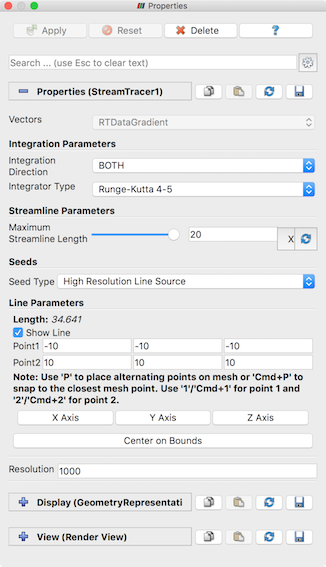Fig. 5.18 The Properties panel showing the default properties for the Stream Tracer filter.

Seeds group lets you set how the seed points for generating the streamlines are produced. You have two options: Point Source , which produces a point clound around the user-specified Point based on the parameters specified, and High Resolution Line Source , which produces seed points along the user-specified line. You can use the 3D widgets shown in the active Render View to interactively place the center for the point cloud or for defining the line.

Did you know?

The Stream Tracer filter produces a polydata with 1D lines for each of the generated streamlines. Since 1D lines cannot be shaded like surfaces in the Render View , you can get visualizations where it is hard to follow the streamlines. To give the streamlines some 3D structure, you can apply the Tube filter to the output of the streamlines. The properties on the Tube filter let you control the thickness of the tubes. You can also vary the thickness of the tubes based on data array, e.g., the magnitude of the vector field at the sample points in the streamline!

A script using the Stream Tracer filter in paraview typically looks like this:

# find source
>>> disk_out_refex2 = FindSource('disk_out_ref.ex2')

# create a new 'Stream Tracer'
>>> streamTracer1 = StreamTracer(Input=disk_out_refex2,
SeedType='Point Source')
>>> streamTracer1.Vectors = ['POINTS', 'V']

# init the 'Point Source' selected for 'SeedType'
>>> streamTracer1.SeedType.Center = [0.0, 0.0, 0.07999992370605469]

# show data in view
>>> Show()

# create a new 'Tube'
>>> tube1 = Tube(Input=streamTracer1)

# Properties modified on tube1

# show the data from tubes in view
>>> Show()


### 5.8.4. Stream Tracer With Custom Source¶

Stream Tracer allows you to specify the seed points either as a point cloud or as a line source. However, if you want to provide your own seed points from another data producer, use the Stream Tracer With Custom Source . Similar to Glyph With Custom Source , this filter allows you to pick a second input connection to use as the seed points.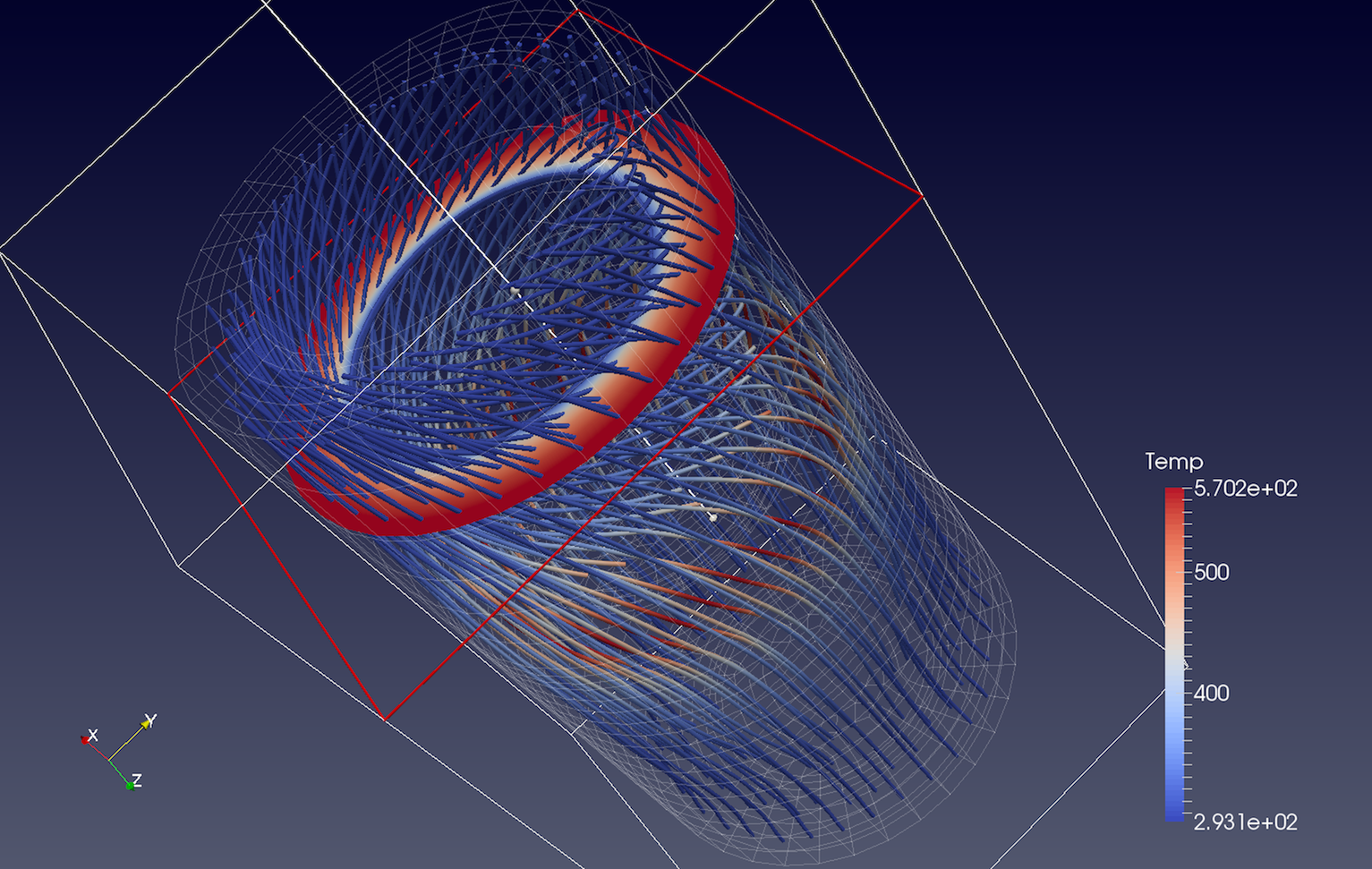Fig. 5.19 Streamlines generated from the disk_out_ref.ex2 dataset using the output of the Slice filter as the Source for seed points.

### 5.8.5. Resample With Dataset¶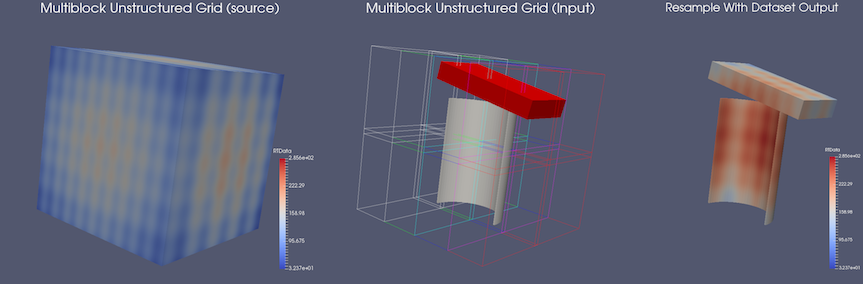Fig. 5.20 An example of Resample With Dataset . On the left is a multiblock tetrahedra mesh ( Input ). The middle shows a multiblock unstructured grid ( Source ). The outline of Input is also shown in this view. The result of applying the filter is shown on the right

Resample With Dataset samples the point and cell attributes of one dataset on to the points of another dataset. The two datasets are supplied to the filter using its two input ports: Input , which is the dataset that provides the attributes to resample, and Source , which is the dataset that provides the points to sample at. This filter is available under the Filters menu.

### 5.8.6. Resample To Image¶

Resample To Image is a specialization of Resample With Dataset . The filter takes one input and samples its point and cell attributes onto a uniform grid of points. The bounds and extents of the uniform grid can be specified using the properties panel. By default, the bounds are set to the bounds of the input dataset. The output of the filter is an Image dataset.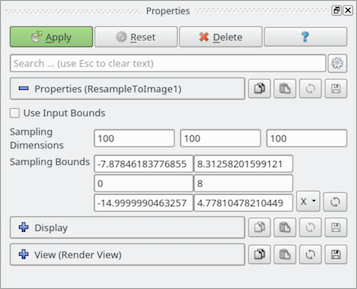Fig. 5.21 The Properties panel for Resample To Image filter.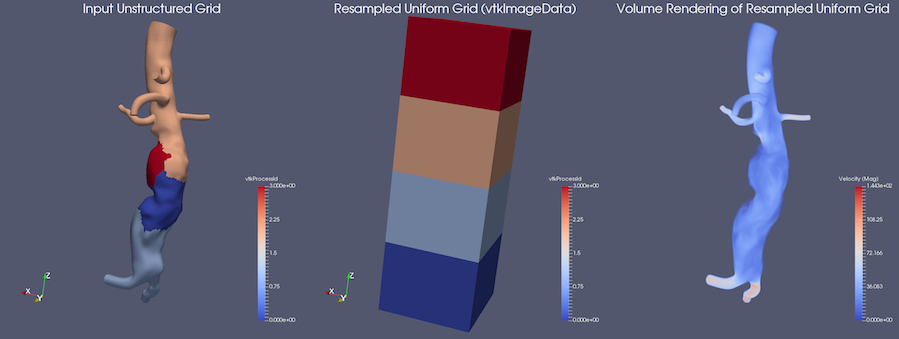Fig. 5.22 An example of Resample To Image . The left portion shows the input (unstructured grid), and the middle displays the output image data. On the right is a volume rendering of the resampled data.

Some operations can be performed more efficiently on uniform grid datasets. Volume rendering is one such operation. The Resample to Image filter can be used to convert any dataset to Image data before performing such operations.

### 5.8.7. Probe¶

Probe samples the input dataset at a specific point location to obtain the cell data attributes for the cell containing the point as well as the interpolated point data attributes. You can either use the SpreadSheet View or the Information panel to inspect the probed values. The probe location can be specified using the interactive 3D widget shown in the active Render View .

### 5.8.8. Plot over line¶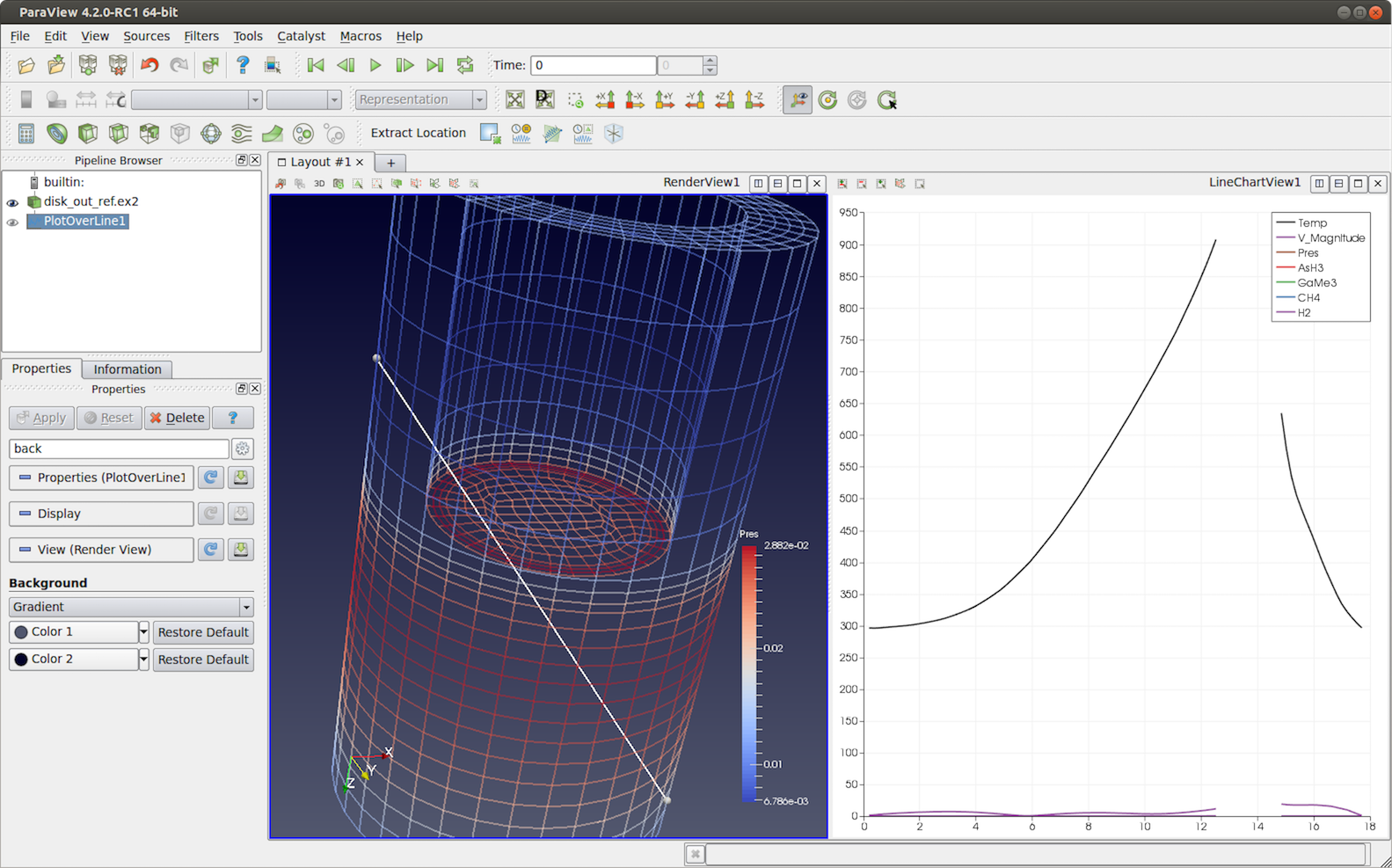Fig. 5.23 The Plot Over Line filter applied to the disk_out_ref.ex2 dataset to plot values at sampled locations along the line. Gaps in the line correspond to the locations in the input dataset where the line falls outside the dataset.

Plot Over Line will sample the input dataset along the specified line and then plot the results in Line Chart View . Internally, this filter uses the same mechanism as the Probe filter, probing along the points in the line to get the containing cell attributes and interpolated point attributes.

Using the Resolution property on the Properties panel, you can control the number of sample points along the line.

## 5.9. Filters for attribute manipulation¶

The filters covered in this section are used to add new attribute arrays to the dataset, which are typically used to add derived quantities to use in pipelines for further processing.

### 5.9.1. Calculator¶

The Calculator filter computes a new data array or new point coordinates as a function of existing input arrays. If point-centered arrays are used in the computation of a new data array, the resulting array will also be point-centered. Similarly, computations using cell-centered arrays will produce a new cell-centered array. If the function is computing point coordinates (requested by checking the Coordinate Results property on the Properties panel) , the result of the function must be a three-component vector. The Calculator interface operates similarly to a scientific calculator. In creating the function to evaluate, the standard order of operations applies. Each of the calculator functions is described below. Unless otherwise noted, enclose the operand in parentheses using the ( and ) buttons.

• Clear : Erase the current function.

• /: Divide one scalar by another. The operands for this function are not required to be enclosed in parentheses.

• *: Multiply two scalars, or multiply a vector by a scalar (scalar multiple). The operands for this function are not required to be enclosed in parentheses.

• -: Negate a scalar or vector (unary minus), or subtract one scalar or vector from another. The operands for this function are not required to be enclosed in parentheses.

• +: Add two scalars or two vectors. The operands for this function are not required to be enclosed in parentheses.

• sin : Compute the sine of a scalar.

• cos : Compute the cosine of a scalar.

• tan : Compute the tangent of a scalar.

• asin : Compute the arcsine of a scalar.

• acos : Compute the arccosine of a scalar.

• atan : Compute the arctangent of a scalar.

• sinh : Compute the hyperbolic sine of a scalar.

• cosh : Compute the hyperbolic cosine of a scalar.

• tanh : Compute the hyperbolic tangent of a scalar.

• min : Compute minimum of two scalars.

• max : Compute maximum of two scalars.

• x^y : Raise one scalar to the power of another scalar. The operands for this function are not required to be enclosed in parentheses.

• sqrt : Compute the square root of a scalar.

• e^x Raise e to the power of a scalar.

• log10 : Compute the logarithm of a scalar to the base 10.

• ln : Compute the logarithm of a scalar to the base $$e$$.

• ceil : Compute the ceiling of a scalar.

• floor : Compute the floor of a scalar.

• abs : Compute the absolute value of a scalar.

• v1.v2 : Compute the dot product of two vectors. The operands for this function are not required to be enclosed in parentheses.

• cross : Compute cross product of two vectors.

• mag : Compute the magnitude of a vector.

• norm : Normalize a vector. The operands are described below. The digits 0-9 and the decimal point are used to enter constant scalar values.

• iHat , jHat , and kHat are vector constants representing unit vectors in the X, Y, and Z directions, respectively.

The Scalars menu lists the names of the scalar arrays and the components of the vector arrays of either the point-centered or cell-centered data. The Vectors menu lists the names of the point-centered or cell-centered vector arrays. The function will be computed for each point (or cell) using the scalar or vector value of the array at that point (or cell). The filter operates on any type of dataset, but the input dataset must have at least one scalar or vector array. The arrays can be either point-centered or cell-centered. The Calculator filter’s output is of the same dataset type as the input.

A common use-case is to convert three input scalars into a vector array. For that, the Function would look something like: $$scalar_x * iHat + scalar_y * jHat + scalar_z * kHat$$.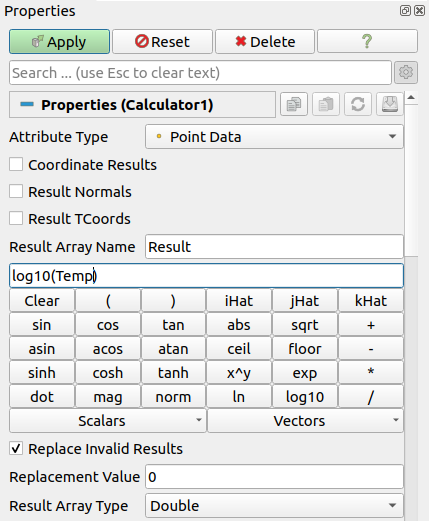Fig. 5.24 The Properties panel for the Calculator filter showing the advanced properties.

The Properties panel provides access to several options for this filter. Checking Coordinate Results , Result Normals , or Result TCoords will set the computed array as the point coordinates, normals, or texture coordinates, respectively. Result Array Name is used to specify a name for the computed array. The default is Result.

Sometimes, the expression can yield invalid values. To replace all invalid values with a specific value, check the Replace Invalid Results checkbox and then enter the value to use to replace invalid values using the Replacement Value . The output array data type is set with the Result Array Type property.

### 5.9.2. Python calculator¶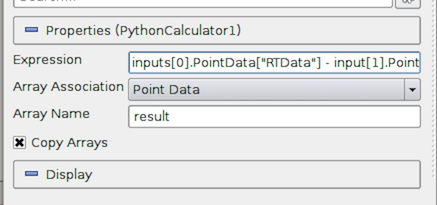Fig. 5.25 The Properties Panel for Python Calculator.

The Python Calculator is similar to Calculator in that it processes one or more input arrays based on an expression provided by the user to produce a new output array. However, it uses Python (and NumPy) to do the computation. Therefore, it provides more expressive expression capabilities.

Specify the Expression to use, the Array Association to indicate the array association ( Point Data or Cell Data ), the name of output array ( Array Name ), and a toggle that controls whether the input arrays are copied to the output ( Copy Array ).

#### 5.9.2.1. Basic tutorial¶

Start by creating a Sphere source and applying the Python Calculator to it. As the first expression, use the following and apply:

5


This should create an array name result in the output point data. Note that this is an array that has a value of 5 for each point. When the expression results in a single value, the calculator will automatically make a constant array. Next, try the following:

Normals


Now, the result array should be the same as the input array Normals. As described in detail later, various functions are available through the calculator. For example, the following is a valid expression:

sin(Normals) + 5


It is very important to note that the Python Calculator has to produce one value per point or cell depending on the Array Association parameter. Most of the functions described here apply individually to all point or cell values and produce an array the same dimensions as the input. However, some of them, such as min() and max() , produce single values.

Common Errors

In the Programmable Filter , all the functions in vtk.numpy_interface.algorithms are imported prior to executing the script. As a result, some built-in functions, such as min and max , are clobbered by that import. To use the built-in functions, import the import __builtin__ module and access those functions with, e.g., __builtin__.min and __builtin__.max

#### 5.9.2.2. Accessing data¶

There are several ways of accessing input arrays within expressions. The simplest way is to access it by name:

sin(Normals) + 5


This is equivalent to:

sin(inputs.PointData['Normals']) + 5


The example above requires some explanation. Here, inputs refer to the first input (dataset) to the filter. Python Calculator can accept multiple inputs. Each input can be accessed as inputs , inputs , … You can access the point or cell data of an input using the .PointData or .CellData qualifiers. You can then access individual arrays within the point or cell data containers using the [] operator. Make sure to use quotes or double-quotes around the array name. Arrays that have names with certain characters (such as space, +, -, *, /) can only be accessed using this method.

Certain functions apply directly on the input mesh. These filters expect an input dataset as argument. For example,

area(inputs)


For data types that explicitly define the point coordinates, you can access the coordinates array using the .Points qualifier. The following extracts the first component of the coordinates array:

inputs.Points[:,0]


Note that for certain data types, mainly image data (uniform rectilinear grids) and rectilinear grids, point coordinates are defined implicitly and cannot be accessed as an array.

#### 5.9.2.3. Comparing multiple datasets¶

The Python Calculator can be used to compare multiple datasets, as shown by the following example.

• Go to the Menu Bar, and select File > Disconnect to clear the Pipeline.

• Select Source > Mandelbrot, and then click Apply, which will set up a default version of the Mandelbrot Set. The data for this set are stored in a $$251 \times 251$$ scalar array.

• Select Source > Mandelbrot again, and then go to the Properties panel and set the Maximum Number of Iterations to 50. Click Apply , which will set up a different version of the Mandelbrot Set, represented by the same size array.

• Hold the Shift key down and select both of the Mandelbrot entries in the Pipeline Inspector, and then go to the Menu Bar, and select Filter > Python Calculator. The two Mandelbrot entries will now be shown as linked, as inputs, to the Python Calculator .

• In the Properties panel for the Python Calculator filter, enter the following into the Expression box:

inputs.PointData['Iterations'] - inputs.PointData['Iterations']


This expression specifies the difference between the second and the first Mandelbrot arrays. The result is saved in a new array called results . The prefixes in the names for the array variables, inputs and inputs , refer to the first and second Mandelbrot entries, respectively, in the Pipeline. PointData specifies that the inputs contain point values. The quoted label 'Iterations' is the local name for these arrays. Click Apply to initiate the calculation.

Click the Display tab in the Properties Panel for the Python Calculator , and go to the first tab to the right of the Color by label. Select the item results in that tab, which will cause the display window to the right to show the results of the expression we entered in the Python Calculator . The scalar values representing the difference between the two Mandelbrot arrays are represented by colors that are set by the current color map (see Edit Color Map… for details).

There are a few things to note:

• Python Calculator will always copy the mesh from the first input to its output.

• All operations are applied point-by-point. In most cases, this requires that the input meshes (topology and geometry) are the same. At the least, it requires that the inputs have the same number of points and cells.

• In parallel execution mode, the inputs have to be distributed exactly the same way across processes.

#### 5.9.2.4. Basic Operations¶

The Python Calculator supports all of the basic arithmetic operations using the $$+$$, $$-$$, $$*$$ and $$/$$ operators. These are always applied element-by-element to point and cell data including scalars, vectors, and tensors. These operations also work with single values. For example, the following adds 5 to all components of all Normals.

Normals + 5


The following adds 1 to the first component, 2 to the second component, and 3 to the third component:

Normals + [1,2,3]


This is specially useful when mixing functions that return single values. For example, the following normalizes the Normals array:

(Normals - min(Normals))/(max(Normals) - min(Normals))


A common use case in a calculator is to work on one component of an array. This can be accomplished with the following:

Normals[:, 0]


The expression above extracts the first component of the Normals vector. Here, : is a placeholder for “all elements”. One element can be extracted by replacing : with an index. For example, the following creates a constant array from the first component of the normal of the first point:

Normals[0, 0]


Alternatively, the following assigns the normal of the first point to all points:

Normals[0, :]


It is also possible to create a vector array from two or three scalar arrays using the make_vector() function:

make_vector(velocity_x, velocity_y, velocity_z)


For temporal datasets, you also have access to the dataset timestep index or time value in the expression as t_index or time_index , and t_value or time_value respectively. When dealing with multiple inputs, you can specify the same variable names scoped on the appropriate input e.g. inputs.t_index .

In some datasets, field data is used to store global data values not associated with cells or points. To use field data in a Python Calculator expression, access it with the FieldData dictionary available in the input as in the following example:

VolumeOfCell * inputs.FieldData['MaterialData'][time_index]


Did you know?

Under the cover, the Python Calculator uses NumPy. All arrays in the expression are compatible with NumPy arrays and can be used where NumPy arrays can be used. For more information on what you can do with these arrays, consult with the NumPy references:cite:numpy.

#### 5.9.2.5. Functions¶

The following is a list of functions available in the Python Calculator . Note that this is a partial list, since most of the NumPy and SciPy functions can be used in the Python Calculator . Many of these functions can take single values or arrays as argument.

• abs(x) : Returns the absolute value(s) of $$x$$.

• add(x, y) : Returns the sum of two values. $$x$$ and $$y$$ can be single values or arrays. This is the same as $$x+y$$.

• area(dataset) : Returns the surface area of each cell in a mesh.

• aspect(dataset) : Returns the aspect ratio of each cell in a mesh.

• aspect_gamma(dataset) : Returns the aspect ratio gamma of each cell in a mesh.

• condition(dataset) : Returns the condition number of each cell in a mesh.

• cross(x, y) : Returns the cross product for two 3D vectors from two arrays of 3D vectors.

• curl(array) : Returns the curl of an array of 3D vectors.

• divergence(array) : Returns the divergence of an array of 3D vectors.

• divide(x, y) : Element-by-element division. $$x$$ and $$y$$ can be single values or arrays. This is the same as math:frac{x}{y}.

• det(array) : Returns the determinant of an array of 2D square matrices.

• determinant(array) : Returns the determinant of an array of 2D square matrices.

• diagonal(dataset) : Returns the diagonal length of each cell in a dataset.

• dot(a1, a2) : Returns the dot product of two scalars/vectors of two array of scalars/vectors.

• eigenvalue(array) : Returns the eigenvalue of an array of 2D square matrices.

• eigenvector(array) : Returns the eigenvector of an array of 2D square matrices.

• exp(x) : Returns $$e^x$$.

• global_max(array) : Returns the maximum value of an array of scalars, vectors, or tensors among all process. This is not yet supported for multi-block and AMR datasets.

• global_mean (array) : Returns the mean value of an array of scalars, vectors, or tensors among all processes. This is not yet supported for multi-block and AMR datasets.

• global_min(array) : Returns the minimum value of an array of scalars/vectors/tensors among all process. This is not yet supported for multi-block and AMR datasets.

• gradient(array) : Returns the gradient of an array of scalars or vectors.

• inv(array) : Returns the inverse an array of 2D square matrices.

• inverse(array) : Returns the inverse of an array of 2D square matrices.

• jacobian(dataset) : Returns the jacobian of an array of 2D square matrices.

• laplacian(array) : Returns the jacobian of an array of scalars.

• ln(array) : Returns the natural logarithm of an array of scalars/vectors/tensors.

• log(array) : Returns the natural logarithm of an array of scalars/vectors/tensors.

• log10(array) : Returns the base 10 logarithm of an array of scalars/vectors/tensors.

• make_point_mask_from_NaNs(dataset, array) : This function will create a ghost array corresponding to an input with NaN values. For each NaN value, the output array will have a corresponding value of vtk.vtkDataSetAttributes.HIDDENPOINT . These values are also combined with any ghost values that the dataset may have.

• make_cell_mask_from_NaNs(dataset, array) : This function will create a ghost array corresponding to an input with NaN values. For each NaN value, the output array will have a corresponding value of vtk.vtkDataSetAttributes.HIDDENCELL . These values are also combined with any ghost values that the dataset may have.

• max(array) : Returns the maximum value of the array as a single value. Note that this function returns the maximum within a block for AMR and multi-block datasets, not across blocks/grids. Also, this returns the maximum within each process when running in parallel.

• max_angle(dataset) : Returns the maximum angle of each cell in a dataset.

• mag(a) : Returns the magnitude of an array of scalars/vectors.

• mean(array) : Returns the mean value of an array of scalars/vectors/tensors.

• min(array) : Returns the minimum value of the array as a single value. Note that this function returns the minimum within a block for AMR and multi-block datasets, not across blocks/grids. Also, this returns the minimum within each process when running in parallel.

• min_angle(dataset) : Returns the minimum angle of each cell in a dataset.

• mod(x, y) : Same as remainder $$(x, y)$$.

• multiply(x, y) : Returns the product of $$x$$ and $$y$$. $$x$$ and $$y$$ can be single values or arrays. Note that this is an element-by-element operation when $$x$$ and $$y$$ are both arrays. This is the same as $$x \times y$$.

• negative(x) : Same as $$-x$$.

• norm(a) : Returns the normalized values of an array of scalars/vectors.

• power(x, a) : Exponentiation of $$x$$ with $$a$$. Here, both $$x$$ and $$a$$ can either be a single value or an array. If $$x$$ and $$y$$ are both arrays, a one-by-one mapping is used between two arrays.

• reciprocal(x) : Returns $$\frac{1}{x}$$.

• remainder(x, y) : Returns $$x - y \times floor(\frac{x}{y})$$. $$x$$ and $$y$$ can be single values or arrays.

• rint(x) : Rounds $$x$$ to the nearest integer(s).

• shear(dataset) : Returns the shear of each cell in a dataset.

• skew(dataset) : Returns the skew of each cell in a dataset.

• square(x) : Returns $$x*x$$.

• sqrt(x) : Returns $$\sqrt{x}$$.

• strain(array) : Returns the strain of an array of 3D vectors.

• subtract(x, y) : Returns the difference between two values. $$x$$ and y can be single values or arrays. This is the same as $$x - y$$.

• surface_normal(dataset) : Returns the surface normal of each cell in a dataset.

• trace(array) : Returns the trace of an array of 2D square matrices.

• volume(dataset) : Returns the volume normal of each cell in a dataset.

• vorticity(array) : Returns the vorticity/curl of an array of 3D vectors.

• vertex_normal(dataset) : Returns the vertex normal of each point in a dataset.

#### 5.9.2.6. Trigonometric Functions¶

Below is a list of supported trigonometric functions:

 sin(x) arccos(x) cosh(x) arctanh(x) cos(x) arctan(x) tanh(x) tan(x) hypot(x1, x2) arcsinh(x) arcsin(x) sinh(x) arccosh(x)

There are two filters that can compute gradients:

• Gradient : This is designed for efficiently computing gradients of point data arrays on a uniform rectilinear grid (Section 3.1.3).

• Gradient of Unstructured DataSet : This computes gradient quantities for a cell or point data array for any type of dataset. This filter optionally computes the gradient, divergence, vorticity which is also known as the curl, and Q-criterion. By default only the gradient computation is enabled. A 3-component array is required in order to compute divergence, vorticity or Q-criterion.

### 5.9.4. Mesh Quality¶

The Mesh Quality filter creates a new cell array containing a geometric measure of each cell’s fitness. Different quality measures can be chosen for different cell shapes.

Triangle Quality indicates which quality measure will be used to evaluate triangle quality. The Radius Ratio is the size of a circle circumscribed by a triangle’s three vertices divided by the size of a circle tangent to a triangle’s three edges. The Edge Ratio is the ratio of the longest edge length to the shortest edge length.

Quad Quality indicates which quality measure will be used to evaluate quad cells.

Tet Quality indicates which quality measure will be used to evaluate tetrahedral quality. The Radius Ratio is the size of a sphere circumscribed by a tetrahedron’s four vertices divided by the size of a circle tangent to a tetrahedron’s four faces. The Edge Ratio is the ratio of the longest edge length to the shortest edge length. The Collapse Ratio is the minimum ratio of height of a vertex above the triangle opposite it, divided by the longest edge of the opposing triangle across all vertex/triangle pairs.

HexQualityMeasure indicates which quality measure will be used to evaluate quality of hexahedral cells.

## 5.10. White-box filters¶

This includes the Programmable Filter and Programmable Source . For these filters/sources, you can add Python code to do the data generation or processing. We’ll cover writing Python code for these in Section 4.

## 5.11. Favorite filters¶

If you use some filters more than others, you can organize them in the Filters > Favorites menu. This can be done from the context menu in the pipeline or through the Filters > Manage Favorites menu as shown in Fig. 5.26. In this dialog you can create categories and subcategories. It supports drag’n’drop operation to sort and move filters and categories. Moreover, Favorites are highlighted in the other filter submenus on supported platforms. Favorites are saved in user settings so they can be used in other subsequent ParaView sessions.Fig. 5.26 The Favorites Manager dialog. Left: the list of available filters. Right: the favorites, organized into categories.

## 5.12. Best practices¶

### 5.12.1. Avoiding data explosion¶

The pipeline model that ParaView presents is very convenient for exploratory visualization. The loose coupling between components provides a very flexible framework for building unique visualizations, and the pipeline structure allows you to tweak parameters quickly and easily.

The downside of this coupling is that it can have a larger memory footprint. Each stage of this pipeline maintains its own copy of the data. Whenever possible, ParaView performs shallow copies of the data so that different stages of the pipeline point to the same block of data in memory. However, any filter that creates new data or changes the values or topology of the data must allocate new memory for the result. If ParaView is filtering a very large mesh, inappropriate use of filters can quickly deplete all available memory. Therefore, when visualizing large datasets, it is important to understand the memory requirements of filters.

Please keep in mind that the following advice is intended only for when dealing with very large amounts of data and the remaining available memory is low. When you are not in danger of running out of memory, the following advice is not relevant.

When dealing with structured data, it is absolutely important to know what filters will change the data to unstructured. Unstructured data has a much higher memory footprint, per cell, than structured data because the topology must be explicitly written out. There are many filters in ParaView that will change the topology in some way, and these filters will write out the data as an unstructured grid, because that is the only dataset that will handle any type of topology that is generated. The following list of filters will write out a new unstructured topology in its output that is roughly equivalent to the input. These filters should never be used with structured data and should be used with caution on unstructured data.

 Append Datasets Extract Edges Subdivide Append Geometry Linear Extrusion Tessellate Clean Loop Subdivision Tetrahedralize Clean to Grid Reflect Triangle Strips Connectivity Rotational Extrusion Triangulate D3 Shrink Delaunay 2D/3D Smooth

Technically, the Ribbon and Tube filters should fall into this list. However, as they only work on 1D cells in poly data, the input data is usually small and of little concern.

This similar set of filters also outputs unstructured grids, but also tends to reduce some of this data. Be aware though that this data reduction is often smaller than the overhead of converting to unstructured data. Also note that the reduction is often not well balanced. It is possible (often likely) that a single process may not lose any cells. Thus, these filters should be used with caution on unstructured data and extreme caution on structured data.

 Clip Extract Selection Decimate Quadric Clustering Extract Cells by Region Threshold

Similar to the items in the preceding list, Extract Subset performs data reduction on a structured dataset, but also outputs a structured dataset. So the warning about creating new data still applies, but you do not have to worry about converting to an unstructured grid.

This next set of filters also outputs unstructured data, but it also performs a reduction on the dimension of the data (for example 3D to 2D), which results in a much smaller output. Thus, these filters are usually safe to use with unstructured data and require only mild caution with structured data.

 Cell Centers Feature Edges Contour Mask Points Extract CTH Fragments Outline (curvilinear) Extract CTH Parts Slice Extract Surface Stream Tracer

The filters below do not change the connectivity of the data at all. Instead, they only add field arrays to the data. All the existing data is shallow copied. These filters are usually safe to use on all data.

 Block Scalars Octree Depth Scalars Calculator Point Data to Cell Data Cell Data to Point Data Process Id Scalars Curvature Random Vectors Elevation Resample with dataset Generate Surface Normals Surface Flow Gradient Surface Vectors Level Scalars Texture Map to… Median Transform Mesh Quality Warp (scalar) Octree Depth Limit Warp (vector)

This final set of filters either add no data to the output (all data of consequence is shallow copied) or the data they add is generally independent of the size of the input. These are almost always safe to add under any circumstances (although they may take a lot of time).

 Annotate Time Outline Append Attributes Outline Corners Extract Block Plot Global Variables Over Time Extract Datasets Plot Over Line Extract Level Plot Selection Over Time Glyph Probe Location Group Datasets Temporal Shift Scale Histogram Temporal Snap-to-Time-Steps Integrate Variables Temporal Statistics Normal Glyphs

There are a few special case filters that do not fit well into any of the previous classes. Some of the filters, currently Temporal Interpolator and Particle Tracer , perform calculations based on how data changes over time. Thus, these filters may need to load data for two or more instances of time, which can double or more the amount of data needed in memory. The Temporal Cache filter will also hold data for multiple instances of time. Keep in mind that some of the temporal filters such as the Temporal Statistics and the filters that plot over time may need to iteratively load all data from disk. Thus, it may take an impractically long amount of time even if does not require any extra memory.

The Programmable Filter is also a special case that is impossible to classify. Since this filter does whatever it is programmed to do, it can fall into any one of these categories.

### 5.12.2. Culling data¶

When dealing with large data, it is best to cull out data whenever possible and do so as early as possible. Most large data starts as 3D geometry and the desired geometry is often a surface. As surfaces usually have a much smaller memory footprint than the volumes that they are derived from, it is best to convert to a surface early on. Once you do that, you can apply other filters in relative safety.

A very common visualization operation is to extract isosurfaces from a volume using the Contour filter. The Contour filter usually outputs geometry much smaller than its input. Thus, the Contour filter should be applied early if it is to be used at all. Be careful when setting up the parameters to the Contour filter because it still is possible for it to generate a lot of data which can happen if you specify many isosurface values. High frequencies such as noise around an isosurface value can also cause a large, irregular surface to form.

Another way to peer inside of a volume is to perform a Slice on it. The Slice filter will intersect a volume with a plane and allow you to see the data in the volume where the plane intersects. If you know the relative location of an interesting feature in your large dataset, slicing is a good way to view it.

If you have little a priori knowledge of your data and would like to explore the data without the long memory and processing time for the full dataset, you can use the Extract Subset filter to subsample the data. The subsampled data can be dramatically smaller than the original data and should still be well load balanced. Of course, be aware that you may miss small features if the subsampling steps over them and that once you find a feature you should go back and visualize it with the full dataset.

There are also several features that can pull out a subset of a volume: Clip , Threshold , Extract Selection , and Extract Subset can all extract cells based on some criterion. Be aware, however, that the extracted cells are almost never well balanced; expect some processes to have no cells removed. All of these filters, with the exception of Extract Subset , will convert structured data types to unstructured grids. Therefore, they should not be used unless the extracted cells are of at least an order of magnitude less than the source data.

When possible, replace the use of a filter that extracts 3D data with one that will extract 2D surfaces. For example, if you are interested in a plane through the data, use the Slice filter rather than the Clip filter. If you are interested in knowing the location of a region of cells containing a particular range of values, consider using the Contour filter to generate surfaces at the ends of the range rather than extract all of the cells with the Threshold filter. Be aware that substituting filters can have an effect on downstream filters. For example, running the Histogram filter after Threshold will have an entirely different effect than running it after the roughly equivalent Contour filter.